## Ellipsoid

A Quadratic Surface which is given in Cartesian Coordinates by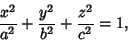(1)

where the semi-axes are of lengths,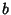, and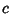. In Spherical Coordinates, this becomes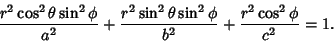(2)

The parametric equations are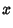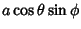(3)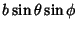(4)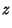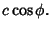(5)

The Surface Area (Bowman 1961, pp. 31-32) is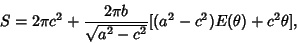(6)

where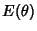is a Complete Elliptic Integral of the Second Kind,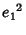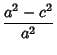(7)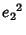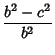(8)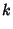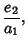(9)

and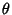is given by inverting the expression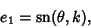(10)

where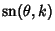is a Jacobi Elliptic Function. The Volume of an ellipsoid is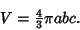(11)

If two axes are the same, the figure is called a Spheroid (depending on whetheror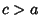, an Oblate Spheroid or Prolate Spheroid, respectively), and if all three are the same, it is a Sphere.

A different parameterization of the ellipsoid is the so-called stereographic ellipsoid, given by the parametric equations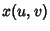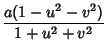(12)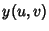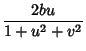(13)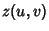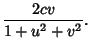(14)

A third parameterization is the Mercator parameterization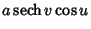(15)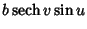(16)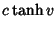(17)

(Gray 1993).

The Support Function of the ellipsoid is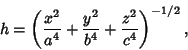(18)

and the Gaussian Curvature is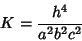(19)

(Gray 1993, p. 296).

References

Beyer, W. H. CRC Standard Mathematical Tables, 28th ed. Boca Raton, FL: CRC Press, p. 131, 1987.

Bowman, F. Introduction to Elliptic Functions, with Applications. New York: Dover, 1961.

Fischer, G. (Ed.). Plate 65 in Mathematische Modelle/Mathematical Models, Bildband/Photograph Volume. Braunschweig, Germany: Vieweg, p. 60, 1986.

Gray, A. The Ellipsoid'' and The Stereographic Ellipsoid.'' §11.2 and 11.3 in Modern Differential Geometry of Curves and Surfaces. Boca Raton, FL: CRC Press, pp. 215-217, and 296, 1993.